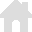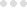vRigger Learning Center

This also applies to cable, chain, and webbing.

Gear that is anchored includes anchors, rocks, trees, tripods, trucks, etc.

A "bight" is a simple loop in a rope that does not cross itself.

A "bend" is a knot that joins two ropes together. Bends can only be attached to the end of a rope.

A "hitch" is a type of knot that must be tied around another object.

"Descending devices" (e.g., ATCs, Brake Bar Racks, Figure 8s, Rescue 8s, etc) create friction as their primary purpose. The friction in descending devices is always considered when calculating forces.

The "Safety Factor" is the ratio between the gear's breaking strength and the maximum load applied to the gear (e.g., 5:1).

# Friction and Force Calculations

## Specifying Friction

Friction is the amount of force that is lost when a rope passes through gear. Learn more about friction at RopeRescueTraining.com.

You can control whether vRigger considers friction by selecting the Consider Friction checkbox in the Calculations group on the Forces toolbar. (The friction in "descending devices" is always considered.)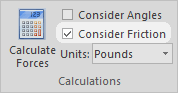When friction is enabled on the toolbar, you can specify the friction of an individual piece of gear in the properties pane.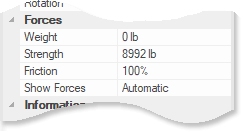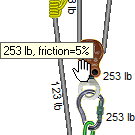When friction is enabled on the toolbar and the forces have been calculated, you can hover your mouse over a piece of gear to display its friction in a ScreenTip. The pulley shown here has 5% friction.

Friction calculations can be confusing. For example, if the pulleys in a typical 3-to-1 haul system each have 10% friction, then the mechanical advantage is closer to 2.7-to-1 rather than 3-to-1. When in doubt, leave friction disabled.

If you change an item's friction, you will need to recalculate the forces.

## Typical friction percentages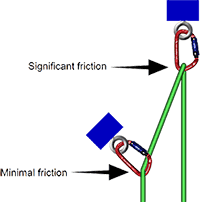As shown on the illustration to the right, the friction generated on a rope is a function of the angle that the rope enters and exists the item. If a rope is barely deflected by a carabiner, it does not generate much friction. If it turns 180° around the biner, it creates significant friction. vRigger does not make this distinction.

## Calculating friction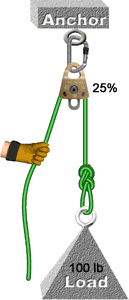When you pull rope through a device with friction, the force is calculated as: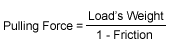When you release rope through a device with friction, the force is calculated as: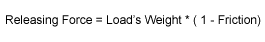The pulley in the example to the right has 25% friction and the load weighs 100 pounds. Using the above formulas, if the hand is pulling, the force on the hand will be 133 pounds (100 divided by 0.75); if the hand is releasing rope, the force on the hand will be 75 pounds (100 multiplied by 0.75).